## Pizza

### Keto Protein Lovers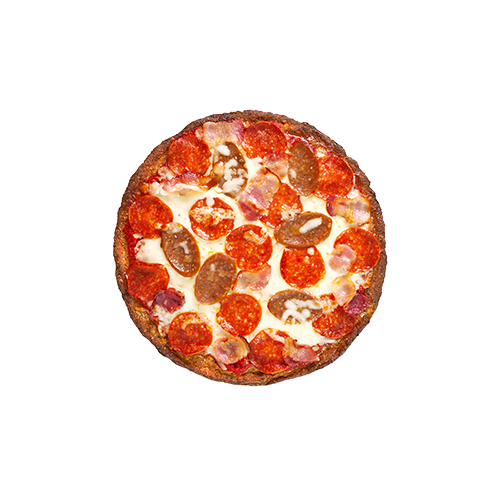### 82

Weight Per Slice (g)

Sugars (g)

Sodium (mg)

Calories (kcals)

Total Fat (g)

Vit A (% DV)

Protein (g)

Sat. Fat (g)

Vit C (% DV)

Carbs (g)

Trans Fat (g)

Calcium (% DV)

Fibre (g)

Cholesterol (mg)

Iron (% DV)

### Keto Pepperoni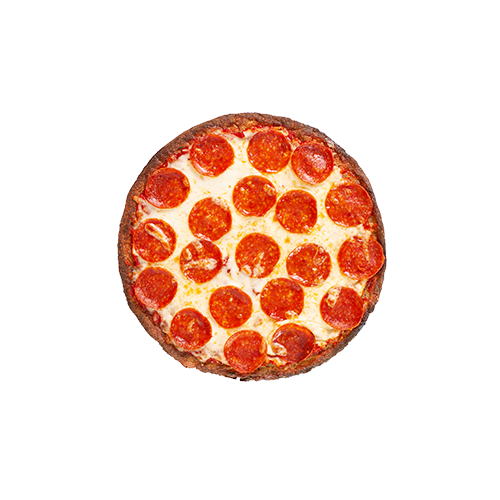### 70

Weight Per Slice (g)

Sugars (g)

Sodium (mg)

Calories (kcals)

Total Fat (g)

Vit A (% DV)

Protein (g)

Sat. Fat (g)

Vit C (% DV)

Carbs (g)

Trans Fat (g)

Calcium (% DV)

Fibre (g)

Cholesterol (mg)

Iron (% DV)

### Keto Pesto Margherita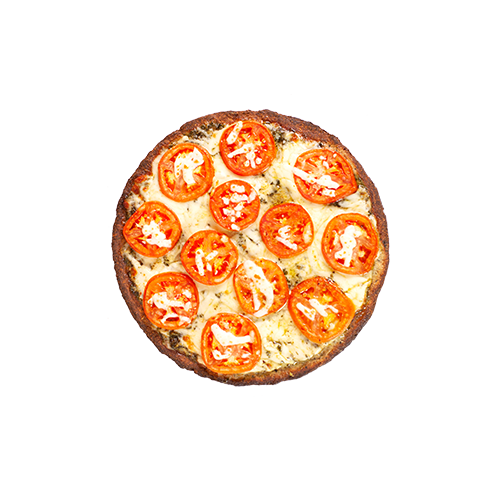### 75

Weight Per Slice (g)

Sugars (g)

Sodium (mg)

Calories (kcals)

Total Fat (g)

Vit A (% DV)

Protein (g)

Sat. Fat (g)

Vit C (% DV)

Carbs (g)

Trans Fat (g)

Calcium (% DV)

Fibre (g)

Cholesterol (mg)

Iron (% DV)

### Everything you need to know about our Low Carb Keto Crust

##### WHAT ARE THE MAIN INGREDIENTS IN THE KETO CRUST?

Water, organic coconut flour, egg whites, liquid whole eggs, organic pumpkin seed flour, psyllium husk, flax meal, extra virgin olive oil, coconut milk, ground chia, salt, apple cider vinegar, garlic powder, cream of tartar, baking soda, Italian herbs, chicory root, yeast.

##### IS THE CRUST GLUTEN FREE?

Yes, the crust is gluten free BUT the keto pizza is prepared in the same area as our regular crust pizzas. For customers that are gluten intolerant or allergic to gluten (i.e. have Celiac disease), we offer Cauliflower Crust Pizzas, Gluten-free Pizzas and Gluten-free Bites.

Disclaimer: Pizza Pizza is a restaurant environment and so, we unfortunately cannot guarantee a 100% allergen-free environment.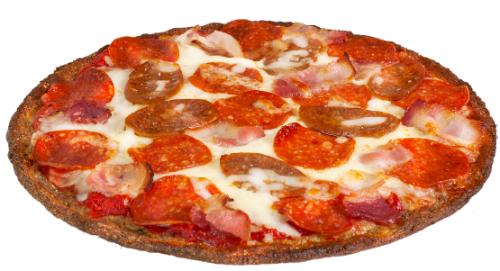##### Keto Protein Lovers

NY Pepperoni, Bacon Strips & Italian Sausage

210
Cals/Slice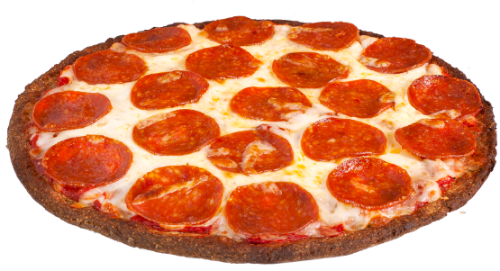##### Keto Pepperoni

NY Pepperoni, Homestyle Tomato Sauce & Mozzarella Cheese

180
Cals/Slice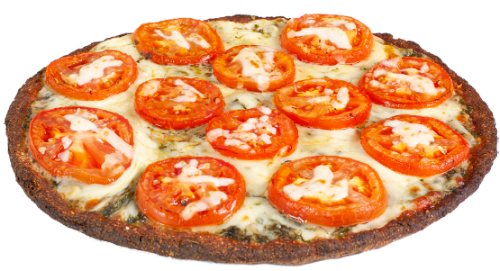##### Keto Pesto Margherita

Pesto Sauce, Roma Tomatoes & Parmesan Cheese

180
Cals/Slice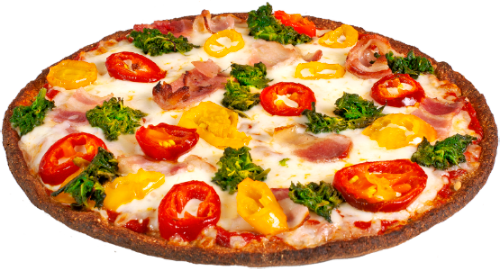##### Build Your Own Keto Pizza

You choose any toppings you want on our Keto Crust

##### IS THE CRUST VEGAN?

No - The crust contains eggs (egg whites and liquid whole eggs) so it is NOT vegan.

##### HOW MANY NET CARBS ARE THERE IN THE KETO CRUST?

4g non-fibre net carbs per crust.

##### WHAT SIZE DOES KETO CRUST COME IN?

Small - 10” with 6 slices.

##### IS THERE ANY ADDED SUGAR IN THE CRUST?

There are no (0g) added sugars in the crust.

##### WHAT ARE THE FAT / PROTEIN / CARB MACROS OF THE CRUST?

Per crust:
Fat = 33g
Protein = 25g
Total Carbohydrates = 32g
Dietary Fibre = 28g

##### WHAT IS THE NAME OF THE SUPPLIER OF THE CRUST?

Keto Crust Supplier - Unbun Foods - unbunfoods.com
Unbun Foods is a Toronto based company that specialises in Keto and Paleo-certified, grain-free products.### 81

Weight Per Slice (g)

Sugars (g)

Sodium (mg)

Calories (kcals)

Total Fat (g)

Vit A (% DV)

Protein (g)

Sat. Fat (g)

Vit C (% DV)

Carbs (g)

Trans Fat (g)

Calcium (% DV)

Fibre (g)

Cholesterol (mg)

Iron (% DV)

### 87

Weight Per Slice (g)

Sugars (g)

Sodium (mg)

Calories (kcals)

Total Fat (g)

Vit A (% DV)

Protein (g)

Sat. Fat (g)

Vit C (% DV)

Carbs (g)

Trans Fat (g)

Calcium (% DV)

Fibre (g)

Cholesterol (mg)

Iron (% DV)

### 92

Weight Per Slice (g)

Sugars (g)

Sodium (mg)

Calories (kcals)

Total Fat (g)

Vit A (% DV)

Protein (g)

Sat. Fat (g)

Vit C (% DV)

Carbs (g)

Trans Fat (g)

Calcium (% DV)

Fibre (g)

Cholesterol (mg)

Iron (% DV)

### Everything you need to know about our Cauliflower Crust

##### WHAT ARE THE INGREDIENTS?

Non-GMO cauliflower, Non-GMO pizza mix (corn starch, rice flour, potato starch, dextrose, vegetable fibers (psyllium, fructose, emulsifier), vegetable fibers (inulin), stabilizer (sorbitol)), water, non-GMO yeast, non-GMO extra virgin olive oil, non-GMO brown cane sugar, non-GMO sea salt.

##### IS THE CRUST GLUTEN FREE?

Yes.

Disclaimer: Pizza Pizza is a restaurant environment and so, we unfortunately cannot guarantee a 100% allergen-free environment.

Yes.

Medium only.

No.

### 91

Weight Per Slice (g)

Sugars (g)

Sodium (mg)

Calories (kcals)

Total Fat (g)

Vit A (% DV)

Protein (g)

Sat. Fat (g)

Vit C (% DV)

Carbs (g)

Trans Fat (g)

Calcium (% DV)

Fibre (g)

Cholesterol (mg)

Iron (% DV)

### 103

Weight Per Slice (g)

Sugars (g)

Sodium (mg)

Calories (kcals)

Total Fat (g)

Vit A (% DV)

Protein (g)

Sat. Fat (g)

Vit C (% DV)

Carbs (g)

Trans Fat (g)

Calcium (% DV)

Fibre (g)

Cholesterol (mg)

Iron (% DV)

### 71

Weight Per Slice (g)

Sugars (g)

Sodium (mg)

Calories (kcals)

Total Fat (g)

Vit A (% DV)

Protein (g)

Sat. Fat (g)

Vit C (% DV)

Carbs (g)

Trans Fat (g)

Calcium (% DV)

Fibre (g)

Cholesterol (mg)

Iron (% DV)

### 85

Weight Per Slice (g)

Sugars (g)

Sodium (mg)

Calories (kcals)

Total Fat (g)

Vit A (% DV)

Protein (g)

Sat. Fat (g)

Vit C (% DV)

Carbs (g)

Trans Fat (g)

Calcium (% DV)

Fibre (g)

Cholesterol (mg)

Iron (% DV)

### 91

Weight Per Slice (g)

Sugars (g)

Sodium (mg)

Calories (kcals)

Total Fat (g)

Vit A (% DV)

Protein (g)

Sat. Fat (g)

Vit C (% DV)

Carbs (g)

Trans Fat (g)

Calcium (% DV)

Fibre (g)

Cholesterol (mg)

Iron (% DV)

### 69

Weight Per Slice (g)

Sugars (g)

Sodium (mg)

Calories (kcals)

Total Fat (g)

Vit A (% DV)

Protein (g)

Sat. Fat (g)

Vit C (% DV)

Carbs (g)

Trans Fat (g)

Calcium (% DV)

Fibre (g)

Cholesterol (mg)

Iron (% DV)

### 76

Weight Per Slice (g)

Sugars (g)

Sodium (mg)

Calories (kcals)

Total Fat (g)

Vit A (% DV)

Protein (g)

Sat. Fat (g)

Vit C (% DV)

Carbs (g)

Trans Fat (g)

Calcium (% DV)

Fibre (g)

Cholesterol (mg)

Iron (% DV)

### 103

Weight Per Slice (g)

Sugars (g)

Sodium (mg)

Calories (kcals)

Total Fat (g)

Vit A (% DV)

Protein (g)

Sat. Fat (g)

Vit C (% DV)

Carbs (g)

Trans Fat (g)

Calcium (% DV)

Fibre (g)

Cholesterol (mg)

Iron (% DV)

### 70

Weight Per Slice (g)

Sugars (g)

Sodium (mg)

Calories (kcals)

Total Fat (g)

Vit A (% DV)

Protein (g)

Sat. Fat (g)

Vit C (% DV)

Carbs (g)

Trans Fat (g)

Calcium (% DV)

Fibre (g)

Cholesterol (mg)

Iron (% DV)

### 76

Weight Per Slice (g)

Sugars (g)

Sodium (mg)

Calories (kcals)

Total Fat (g)

Vit A (% DV)

Protein (g)

Sat. Fat (g)

Vit C (% DV)

Carbs (g)

Trans Fat (g)

Calcium (% DV)

Fibre (g)

Cholesterol (mg)

### 2%

Iron (% DV)

[/vc_column_text]

### Everything you need to know about our Gluten-Free Crust

##### WHAT ARE THE INGREDIENTS?
Rice Flour, Filtered Water, Tapioca Starch, Potato Starch, Extra Virgin Olive Oil, Palm and Soybean Oils, Sugar, Modified Corn Starch, Yeast, Potassium Chloride, Salt, Modified Cellulose, Xanthan Gum, Natural Flavor, Sodium Bicarbonate, Sodium Aluminum Phosphate, Distilled Monoglycerides, Guar Gum.

##### IS THE CRUST GLUTEN FREE?

Yes.

Disclaimer: Pizza Pizza is a restaurant environment and so, we unfortunately cannot guarantee a 100% allergen-free environment.

Yes.

Small only.

No.

## Meat Favourites

### 105

Weight Per Slice (g)

Sugars (g)

Sodium (mg)

Calories (kcals)

Total Fat (g)

Vit A (% DV)

Protein (g)

Sat. Fat (g)

Vit C (% DV)

Carbs (g)

Trans Fat (g)

Calcium (% DV)

Fibre (g)

Cholesterol (mg)

Iron (% DV)

### 95

Weight Per Slice (g)

Sugars (g)

Sodium (mg)

Calories (kcals)

Total Fat (g)

Vit A (% DV)

Protein (g)

Sat. Fat (g)

Vit C (% DV)

Carbs (g)

Trans Fat (g)

Calcium (% DV)

Fibre (g)

Cholesterol (mg)

Iron (% DV)

### 100

Weight Per Slice (g)

Sugars (g)

Sodium (mg)

Calories (kcals)

Total Fat (g)

Vit A (% DV)

Protein (g)

Sat. Fat (g)

Vit C (% DV)

Carbs (g)

Trans Fat (g)

Calcium (% DV)

Fibre (g)

Cholesterol (mg)

Iron (% DV)

### 103

Weight Per Slice (g)

Sugars (g)

Sodium (mg)

Calories (kcals)

Total Fat (g)

Vit A (% DV)

Protein (g)

Sat. Fat (g)

Vit C (% DV)

Carbs (g)

Trans Fat (g)

Calcium (% DV)

Fibre (g)

Cholesterol (mg)

Iron (% DV)

### 107

Weight Per Slice (g)

Sugars (g)

Sodium (mg)

Calories (kcals)

Total Fat (g)

Vit A (% DV)

Protein (g)

Sat. Fat (g)

Vit C (% DV)

Carbs (g)

Trans Fat (g)

Calcium (% DV)

Fibre (g)

Cholesterol (mg)

Iron (% DV)

### 93

Weight Per Slice (g)

Sugars (g)

Sodium (mg)

Calories (kcals)

Total Fat (g)

Vit A (% DV)

Protein (g)

Sat. Fat (g)

Vit C (% DV)

Carbs (g)

Trans Fat (g)

Calcium (% DV)

Fibre (g)

Cholesterol (mg)

Iron (% DV)

### 93

Weight Per Slice (g)

Sugars (g)

Sodium (mg)

Calories (kcals)

Total Fat (g)

Vit A (% DV)

Protein (g)

Sat. Fat (g)

Vit C (% DV)

Carbs (g)

Trans Fat (g)

Calcium (% DV)

Fibre (g)

Cholesterol (mg)

Iron (% DV)

### 101

Weight Per Slice (g)

Sugars (g)

Sodium (mg)

Calories (kcals)

Total Fat (g)

Vit A (% DV)

Protein (g)

Sat. Fat (g)

Vit C (% DV)

Carbs (g)

Trans Fat (g)

Calcium (% DV)

Fibre (g)

Cholesterol (mg)

Iron (% DV)

### 81

Weight Per Slice (g)

Sugars (g)

Sodium (mg)

Calories (kcals)

Total Fat (g)

Vit A (% DV)

Protein (g)

Sat. Fat (g)

Vit C (% DV)

Carbs (g)

Trans Fat (g)

Calcium (% DV)

Fibre (g)

Cholesterol (mg)

Iron (% DV)

### 94

Weight Per Slice (g)

Sugars (g)

Sodium (mg)

Calories (kcals)

Total Fat (g)

Vit A (% DV)

Protein (g)

Sat. Fat (g)

Vit C (% DV)

Carbs (g)

Trans Fat (g)

Calcium (% DV)

Fibre (g)

Cholesterol (mg)

Iron (% DV)

### 107

Weight Per Slice (g)

Sugars (g)

Sodium (mg)

Calories (kcals)

Total Fat (g)

Vit A (% DV)

Protein (g)

Sat. Fat (g)

Vit C (% DV)

Carbs (g)

Trans Fat (g)

Calcium (% DV)

Fibre (g)

Cholesterol (mg)

Iron (% DV)

### 87

Weight Per Slice (g)

Sugars (g)

Sodium (mg)

Calories (kcals)

Total Fat (g)

Vit A (% DV)

Protein (g)

Sat. Fat (g)

Vit C (% DV)

Carbs (g)

Trans Fat (g)

Calcium (% DV)

Fibre (g)

Cholesterol (mg)

Iron (% DV)

### 92

Weight Per Slice (g)

Sugars (g)

Sodium (mg)

Calories (kcals)

Total Fat (g)

Vit A (% DV)

Protein (g)

Sat. Fat (g)

Vit C (% DV)

Carbs (g)

Trans Fat (g)

Calcium (% DV)

Fibre (g)

Cholesterol (mg)

Iron (% DV)

### 109

Weight Per Slice (g)

Sugars (g)

Sodium (mg)

Calories (kcals)

Total Fat (g)

Vit A (% DV)

Protein (g)

Sat. Fat (g)

Vit C (% DV)

Carbs (g)

Trans Fat (g)

Calcium (% DV)

Fibre (g)

Cholesterol (mg)

Iron (% DV)

### 93

Weight Per Slice (g)

Sugars (g)

Sodium (mg)

Calories (kcals)

Total Fat (g)

Vit A (% DV)

Protein (g)

Sat. Fat (g)

Vit C (% DV)

Carbs (g)

Trans Fat (g)

Calcium (% DV)

Fibre (g)

Cholesterol (mg)

Iron (% DV)

### 105

Weight Per Slice (g)

Sugars (g)

Sodium (mg)

Calories (kcals)

Total Fat (g)

Vit A (% DV)

Protein (g)

Sat. Fat (g)

Vit C (% DV)

Carbs (g)

Trans Fat (g)

Calcium (% DV)

Fibre (g)

Cholesterol (mg)

Iron (% DV)

### 102

Weight Per Slice (g)

Sugars (g)

Sodium (mg)

Calories (kcals)

Total Fat (g)

Vit A (% DV)

Protein (g)

Sat. Fat (g)

Vit C (% DV)

Carbs (g)

Trans Fat (g)

Calcium (% DV)

Fibre (g)

Cholesterol (mg)

Iron (% DV)

### 89

Weight Per Slice (g)

Sugars (g)

Sodium (mg)

Calories (kcals)

Total Fat (g)

Vit A (% DV)

Protein (g)

Sat. Fat (g)

Vit C (% DV)

Carbs (g)

Trans Fat (g)

Calcium (% DV)

Fibre (g)

Cholesterol (mg)

Iron (% DV)

### 99

Weight Per Slice (g)

Sugars (g)

Sodium (mg)

Calories (kcals)

Total Fat (g)

Vit A (% DV)

Protein (g)

Sat. Fat (g)

Vit C (% DV)

Carbs (g)

Trans Fat (g)

Calcium (% DV)

Fibre (g)

Cholesterol (mg)

Iron (% DV)

## Veggie Favourites

### 76

Weight Per Slice (g)

Sugars (g)

Sodium (mg)

Calories (kcals)

Total Fat (g)

Vit A (% DV)

Protein (g)

Sat. Fat (g)

Vit C (% DV)

Carbs (g)

Trans Fat (g)

Calcium (% DV)

Fibre (g)

Cholesterol (mg)

Iron (% DV)

### 107

Weight Per Slice (g)

Sugars (g)

Sodium (mg)

Calories (kcals)

Total Fat (g)

Vit A (% DV)

Protein (g)

Sat. Fat (g)

Vit C (% DV)

Carbs (g)

Trans Fat (g)

Calcium (% DV)

Fibre (g)

Cholesterol (mg)

Iron (% DV)

### 95

Weight Per Slice (g)

Sugars (g)

Sodium (mg)

Calories (kcals)

Total Fat (g)

Vit A (% DV)

Protein (g)

Sat. Fat (g)

Vit C (% DV)

Carbs (g)

Trans Fat (g)

Calcium (% DV)

Fibre (g)

Cholesterol (mg)

Iron (% DV)

### 102

Weight Per Slice (g)

Sugars (g)

Sodium (mg)

Calories (kcals)

Total Fat (g)

Vit A (% DV)

Protein (g)

Sat. Fat (g)

Vit C (% DV)

Carbs (g)

Trans Fat (g)

Calcium (% DV)

Fibre (g)

Cholesterol (mg)

Iron (% DV)

### 112

Weight Per Slice (g)

Sugars (g)

Sodium (mg)

Calories (kcals)

Total Fat (g)

Vit A (% DV)

Protein (g)

Sat. Fat (g)

Vit C (% DV)

Carbs (g)

Trans Fat (g)

Calcium (% DV)

Fibre (g)

Cholesterol (mg)

Iron (% DV)

### 95

Weight Per Slice (g)

Sugars (g)

Sodium (mg)

Calories (kcals)

Total Fat (g)

Vit A (% DV)

Protein (g)

Sat. Fat (g)

Vit C (% DV)

Carbs (g)

Trans Fat (g)

Calcium (% DV)

Fibre (g)

Cholesterol (mg)

Iron (% DV)

### 84

Weight Per Slice (g)

Sugars (g)

Sodium (mg)

Calories (kcals)

Total Fat (g)

Vit A (% DV)

Protein (g)

Sat. Fat (g)

Vit C (% DV)

Carbs (g)

Trans Fat (g)

Calcium (% DV)

Fibre (g)

Cholesterol (mg)

Iron (% DV)

### 98

Weight Per Slice (g)

Sugars (g)

Sodium (mg)

Calories (kcals)

Total Fat (g)

Vit A (% DV)

Protein (g)

Sat. Fat (g)

Vit C (% DV)

Carbs (g)

Trans Fat (g)

Calcium (% DV)

Fibre (g)

Cholesterol (mg)

Iron (% DV)

## Meat Favourites

### 111

Weight Per Slice (g)

Sugars (g)

Sodium (mg)

Calories (kcals)

Total Fat (g)

Vit A (% DV)

Protein (g)

Sat. Fat (g)

Vit C (% DV)

Carbs (g)

Trans Fat (g)

Calcium (% DV)

Fibre (g)

Cholesterol (mg)

Iron (% DV)

### 96

Weight Per Slice (g)

Sugars (g)

Sodium (mg)

Calories (kcals)

Total Fat (g)

Vit A (% DV)

Protein (g)

Sat. Fat (g)

Vit C (% DV)

Carbs (g)

Trans Fat (g)

Calcium (% DV)

Fibre (g)

Cholesterol (mg)

Iron (% DV)

### 103

Weight Per Slice (g)

Sugars (g)

Sodium (mg)

Calories (kcals)

Total Fat (g)

Vit A (% DV)

Protein (g)

Sat. Fat (g)

Vit C (% DV)

Carbs (g)

Trans Fat (g)

Calcium (% DV)

Fibre (g)

Cholesterol (mg)

Iron (% DV)

### 110

Weight Per Slice (g)

Sugars (g)

Sodium (mg)

Calories (kcals)

Total Fat (g)

Vit A (% DV)

Protein (g)

Sat. Fat (g)

Vit C (% DV)

Carbs (g)

Trans Fat (g)

Calcium (% DV)

Fibre (g)

Cholesterol (mg)

Iron (% DV)

### 110

Weight Per Slice (g)

Sugars (g)

Sodium (mg)

Calories (kcals)

Total Fat (g)

Vit A (% DV)

Protein (g)

Sat. Fat (g)

Vit C (% DV)

Carbs (g)

Trans Fat (g)

Calcium (% DV)

Fibre (g)

Cholesterol (mg)

Iron (% DV)

### 96

Weight Per Slice (g)

Sugars (g)

Sodium (mg)

Calories (kcals)

Total Fat (g)

Vit A (% DV)

Protein (g)

Sat. Fat (g)

Vit C (% DV)

Carbs (g)

Trans Fat (g)

Calcium (% DV)

Fibre (g)

Cholesterol (mg)

Iron (% DV)

### 96

Weight Per Slice (g)

Sugars (g)

Sodium (mg)

Calories (kcals)

Total Fat (g)

Vit A (% DV)

Protein (g)

Sat. Fat (g)

Vit C (% DV)

Carbs (g)

Trans Fat (g)

Calcium (% DV)

Fibre (g)

Cholesterol (mg)

Iron (% DV)

### 108

Weight Per Slice (g)

Sugars (g)

Sodium (mg)

Calories (kcals)

Total Fat (g)

Vit A (% DV)

Protein (g)

Sat. Fat (g)

Vit C (% DV)

Carbs (g)

Trans Fat (g)

Calcium (% DV)

Fibre (g)

Cholesterol (mg)

Iron (% DV)

### 82

Weight Per Slice (g)

Sugars (g)

Sodium (mg)

Calories (kcals)

Total Fat (g)

Vit A (% DV)

Protein (g)

Sat. Fat (g)

Vit C (% DV)

Carbs (g)

Trans Fat (g)

Calcium (% DV)

Fibre (g)

Cholesterol (mg)

Iron (% DV)

### 98

Weight Per Slice (g)

Sugars (g)

Sodium (mg)

Calories (kcals)

Total Fat (g)

Vit A (% DV)

Protein (g)

Sat. Fat (g)

Vit C (% DV)

Carbs (g)

Trans Fat (g)

Calcium (% DV)

Fibre (g)

Cholesterol (mg)

Iron (% DV)

### 112

Weight Per Slice (g)

Sugars (g)

Sodium (mg)

Calories (kcals)

Total Fat (g)

Vit A (% DV)

Protein (g)

Sat. Fat (g)

Vit C (% DV)

Carbs (g)

Trans Fat (g)

Calcium (% DV)

Fibre (g)

Cholesterol (mg)

Iron (% DV)

### 88

Weight Per Slice (g)

Sugars (g)

Sodium (mg)

Calories (kcals)

Total Fat (g)

Vit A (% DV)

Protein (g)

Sat. Fat (g)

Vit C (% DV)

Carbs (g)

Trans Fat (g)

Calcium (% DV)

Fibre (g)

Cholesterol (mg)

Iron (% DV)

### 98

Weight Per Slice (g)

Sugars (g)

Sodium (mg)

Calories (kcals)

Total Fat (g)

Vit A (% DV)

Protein (g)

Sat. Fat (g)

Vit C (% DV)

Carbs (g)

Trans Fat (g)

Calcium (% DV)

Fibre (g)

Cholesterol (mg)

Iron (% DV)

### 112

Weight Per Slice (g)

Sugars (g)

Sodium (mg)

Calories (kcals)

Total Fat (g)

Vit A (% DV)

Protein (g)

Sat. Fat (g)

Vit C (% DV)

Carbs (g)

Trans Fat (g)

Calcium (% DV)

Fibre (g)

Cholesterol (mg)

Iron (% DV)

### 99

Weight Per Slice (g)

Sugars (g)

Sodium (mg)

Calories (kcals)

Total Fat (g)

Vit A (% DV)

Protein (g)

Sat. Fat (g)

Vit C (% DV)

Carbs (g)

Trans Fat (g)

Calcium (% DV)

Fibre (g)

Cholesterol (mg)

Iron (% DV)

### 111

Weight Per Slice (g)

Sugars (g)

Sodium (mg)

Calories (kcals)

Total Fat (g)

Vit A (% DV)

Protein (g)

Sat. Fat (g)

Vit C (% DV)

Carbs (g)

Trans Fat (g)

Calcium (% DV)

Fibre (g)

Cholesterol (mg)

Iron (% DV)

### 105

Weight Per Slice (g)

Sugars (g)

Sodium (mg)

Calories (kcals)

Total Fat (g)

Vit A (% DV)

Protein (g)

Sat. Fat (g)

Vit C (% DV)

Carbs (g)

Trans Fat (g)

Calcium (% DV)

Fibre (g)

Cholesterol (mg)

Iron (% DV)

### 92

Weight Per Slice (g)

Sugars (g)

Sodium (mg)

Calories (kcals)

Total Fat (g)

Vit A (% DV)

Protein (g)

Sat. Fat (g)

Vit C (% DV)

Carbs (g)

Trans Fat (g)

Calcium (% DV)

Fibre (g)

Cholesterol (mg)

Iron (% DV)

### 101

Weight Per Slice (g)

Sugars (g)

Sodium (mg)

Calories (kcals)

Total Fat (g)

Vit A (% DV)

Protein (g)

Sat. Fat (g)

Vit C (% DV)

Carbs (g)

Trans Fat (g)

Calcium (% DV)

Fibre (g)

Cholesterol (mg)

Iron (% DV)

## Veggie Favourites

### 77

Weight Per Slice (g)

Sugars (g)

Sodium (mg)

Calories (kcals)

Total Fat (g)

Vit A (% DV)

Protein (g)

Sat. Fat (g)

Vit C (% DV)

Carbs (g)

Trans Fat (g)

Calcium (% DV)

Fibre (g)

Cholesterol (mg)

Iron (% DV)

### 111

Weight Per Slice (g)

Sugars (g)

Sodium (mg)

Calories (kcals)

Total Fat (g)

Vit A (% DV)

Protein (g)

Sat. Fat (g)

Vit C (% DV)

Carbs (g)

Trans Fat (g)

Calcium (% DV)

Fibre (g)

Cholesterol (mg)

Iron (% DV)

### 98

Weight Per Slice (g)

Sugars (g)

Sodium (mg)

Calories (kcals)

Total Fat (g)

Vit A (% DV)

Protein (g)

Sat. Fat (g)

Vit C (% DV)

Carbs (g)

Trans Fat (g)

Calcium (% DV)

Fibre (g)

Cholesterol (mg)

Iron (% DV)

### 105

Weight Per Slice (g)

Sugars (g)

Sodium (mg)

Calories (kcals)

Total Fat (g)

Vit A (% DV)

Protein (g)

Sat. Fat (g)

Vit C (% DV)

Carbs (g)

Trans Fat (g)

Calcium (% DV)

Fibre (g)

Cholesterol (mg)

Iron (% DV)

### 118

Weight Per Slice (g)

Sugars (g)

Sodium (mg)

Calories (kcals)

Total Fat (g)

Vit A (% DV)

Protein (g)

Sat. Fat (g)

Vit C (% DV)

Carbs (g)

Trans Fat (g)

Calcium (% DV)

Fibre (g)

Cholesterol (mg)

Iron (% DV)

### 98

Weight Per Slice (g)

Sugars (g)

Sodium (mg)

Calories (kcals)

Total Fat (g)

Vit A (% DV)

Protein (g)

Sat. Fat (g)

Vit C (% DV)

Carbs (g)

Trans Fat (g)

Calcium (% DV)

Fibre (g)

Cholesterol (mg)

Iron (% DV)

### 86

Weight Per Slice (g)

Sugars (g)

Sodium (mg)

Calories (kcals)

Total Fat (g)

Vit A (% DV)

Protein (g)

Sat. Fat (g)

Vit C (% DV)

Carbs (g)

Trans Fat (g)

Calcium (% DV)

Fibre (g)

Cholesterol (mg)

Iron (% DV)

### 102

Weight Per Slice (g)

Sugars (g)

Sodium (mg)

Calories (kcals)

Total Fat (g)

Vit A (% DV)

Protein (g)

Sat. Fat (g)

Vit C (% DV)

Carbs (g)

Trans Fat (g)

Calcium (% DV)

Fibre (g)

Cholesterol (mg)

Iron (% DV)

## Meat Favourites

### 123

Weight Per Slice (g)

Sugars (g)

Sodium (mg)

Calories (kcals)

Total Fat (g)

Vit A (% DV)

Protein (g)

Sat. Fat (g)

Vit C (% DV)

Carbs (g)

Trans Fat (g)

Calcium (% DV)

Fibre (g)

Cholesterol (mg)

Iron (% DV)

### 107

Weight Per Slice (g)

Sugars (g)

Sodium (mg)

Calories (kcals)

Total Fat (g)

Vit A (% DV)

Protein (g)

Sat. Fat (g)

Vit C (% DV)

Carbs (g)

Trans Fat (g)

Calcium (% DV)

Fibre (g)

Cholesterol (mg)

Iron (% DV)

### 117

Weight Per Slice (g)

Sugars (g)

Sodium (mg)

Calories (kcals)

Total Fat (g)

Vit A (% DV)

Protein (g)

Sat. Fat (g)

Vit C (% DV)

Carbs (g)

Trans Fat (g)

Calcium (% DV)

Fibre (g)

Cholesterol (mg)

Iron (% DV)

### 120

Weight Per Slice (g)

Sugars (g)

Sodium (mg)

Calories (kcals)

Total Fat (g)

Vit A (% DV)

Protein (g)

Sat. Fat (g)

Vit C (% DV)

Carbs (g)

Trans Fat (g)

Calcium (% DV)

Fibre (g)

Cholesterol (mg)

Iron (% DV)

### 122

Weight Per Slice (g)

Sugars (g)

Sodium (mg)

Calories (kcals)

Total Fat (g)

Vit A (% DV)

Protein (g)

Sat. Fat (g)

Vit C (% DV)

Carbs (g)

Trans Fat (g)

Calcium (% DV)

Fibre (g)

Cholesterol (mg)

Iron (% DV)

### 109

Weight Per Slice (g)

Sugars (g)

Sodium (mg)

Calories (kcals)

Total Fat (g)

Vit A (% DV)

Protein (g)

Sat. Fat (g)

Vit C (% DV)

Carbs (g)

Trans Fat (g)

Calcium (% DV)

Fibre (g)

Cholesterol (mg)

Iron (% DV)

### 109

Weight Per Slice (g)

Sugars (g)

Sodium (mg)

Calories (kcals)

Total Fat (g)

Vit A (% DV)

Protein (g)

Sat. Fat (g)

Vit C (% DV)

Carbs (g)

Trans Fat (g)

Calcium (% DV)

Fibre (g)

Cholesterol (mg)

Iron (% DV)

### 120

Weight Per Slice (g)

Sugars (g)

Sodium (mg)

Calories (kcals)

Total Fat (g)

Vit A (% DV)

Protein (g)

Sat. Fat (g)

Vit C (% DV)

Carbs (g)

Trans Fat (g)

Calcium (% DV)

Fibre (g)

Cholesterol (mg)

Iron (% DV)

### 97

Weight Per Slice (g)

Sugars (g)

Sodium (mg)

Calories (kcals)

Total Fat (g)

Vit A (% DV)

Protein (g)

Sat. Fat (g)

Vit C (% DV)

Carbs (g)

Trans Fat (g)

Calcium (% DV)

Fibre (g)

Cholesterol (mg)

Iron (% DV)

### 110

Weight Per Slice (g)

Sugars (g)

Sodium (mg)

Calories (kcals)

Total Fat (g)

Vit A (% DV)

Protein (g)

Sat. Fat (g)

Vit C (% DV)

Carbs (g)

Trans Fat (g)

Calcium (% DV)

Fibre (g)

Cholesterol (mg)

Iron (% DV)

### 124

Weight Per Slice (g)

Sugars (g)

Sodium (mg)

Calories (kcals)

Total Fat (g)

Vit A (% DV)

Protein (g)

Sat. Fat (g)

Vit C (% DV)

Carbs (g)

Trans Fat (g)

Calcium (% DV)

Fibre (g)

Cholesterol (mg)

Iron (% DV)

### 100

Weight Per Slice (g)

Sugars (g)

Sodium (mg)

Calories (kcals)

Total Fat (g)

Vit A (% DV)

Protein (g)

Sat. Fat (g)

Vit C (% DV)

Carbs (g)

Trans Fat (g)

Calcium (% DV)

Fibre (g)

Cholesterol (mg)

Iron (% DV)

### 111

Weight Per Slice (g)

Sugars (g)

Sodium (mg)

Calories (kcals)

Total Fat (g)

Vit A (% DV)

Protein (g)

Sat. Fat (g)

Vit C (% DV)

Carbs (g)

Trans Fat (g)

Calcium (% DV)

Fibre (g)

Cholesterol (mg)

Iron (% DV)

### 124

Weight Per Slice (g)

Sugars (g)

Sodium (mg)

Calories (kcals)

Total Fat (g)

Vit A (% DV)

Protein (g)

Sat. Fat (g)

Vit C (% DV)

Carbs (g)

Trans Fat (g)

Calcium (% DV)

Fibre (g)

Cholesterol (mg)

Iron (% DV)

### 110

Weight Per Slice (g)

Sugars (g)

Sodium (mg)

Calories (kcals)

Total Fat (g)

Vit A (% DV)

Protein (g)

Sat. Fat (g)

Vit C (% DV)

Carbs (g)

Trans Fat (g)

Calcium (% DV)

Fibre (g)

Cholesterol (mg)

Iron (% DV)

### 124

Weight Per Slice (g)

Sugars (g)

Sodium (mg)

Calories (kcals)

Total Fat (g)

Vit A (% DV)

Protein (g)

Sat. Fat (g)

Vit C (% DV)

Carbs (g)

Trans Fat (g)

Calcium (% DV)

Fibre (g)

Cholesterol (mg)

Iron (% DV)

### 117

Weight Per Slice (g)

Sugars (g)

Sodium (mg)

Calories (kcals)

Total Fat (g)

Vit A (% DV)

Protein (g)

Sat. Fat (g)

Vit C (% DV)

Carbs (g)

Trans Fat (g)

Calcium (% DV)

Fibre (g)

Cholesterol (mg)

Iron (% DV)

### 103

Weight Per Slice (g)

Sugars (g)

Sodium (mg)

Calories (kcals)

Total Fat (g)

Vit A (% DV)

Protein (g)

Sat. Fat (g)

Vit C (% DV)

Carbs (g)

Trans Fat (g)

Calcium (% DV)

Fibre (g)

Cholesterol (mg)

Iron (% DV)

### 113

Weight Per Slice (g)

Sugars (g)

Sodium (mg)

Calories (kcals)

Total Fat (g)

Vit A (% DV)

Protein (g)

Sat. Fat (g)

Vit C (% DV)

Carbs (g)

Trans Fat (g)

Calcium (% DV)

Fibre (g)

Cholesterol (mg)

Iron (% DV)

## Veggie Favourites

### 90

Weight Per Slice (g)

Sugars (g)

Sodium (mg)

Calories (kcals)

Total Fat (g)

Vit A (% DV)

Protein (g)

Sat. Fat (g)

Vit C (% DV)

Carbs (g)

Trans Fat (g)

Calcium (% DV)

Fibre (g)

Cholesterol (mg)

Iron (% DV)

### 123

Weight Per Slice (g)

Sugars (g)

Sodium (mg)

Calories (kcals)

Total Fat (g)

Vit A (% DV)

Protein (g)

Sat. Fat (g)

Vit C (% DV)

Carbs (g)

Trans Fat (g)

Calcium (% DV)

Fibre (g)

Cholesterol (mg)

Iron (% DV)

### 110

Weight Per Slice (g)

Sugars (g)

Sodium (mg)

Calories (kcals)

Total Fat (g)

Vit A (% DV)

Protein (g)

Sat. Fat (g)

Vit C (% DV)

Carbs (g)

Trans Fat (g)

Calcium (% DV)

Fibre (g)

Cholesterol (mg)

Iron (% DV)

### 119

Weight Per Slice (g)

Sugars (g)

Sodium (mg)

Calories (kcals)

Total Fat (g)

Vit A (% DV)

Protein (g)

Sat. Fat (g)

Vit C (% DV)

Carbs (g)

Trans Fat (g)

Calcium (% DV)

Fibre (g)

Cholesterol (mg)

Iron (% DV)

### 133

Weight Per Slice (g)

Sugars (g)

Sodium (mg)

Calories (kcals)

Total Fat (g)

Vit A (% DV)

Protein (g)

Sat. Fat (g)

Vit C (% DV)

Carbs (g)

Trans Fat (g)

Calcium (% DV)

Fibre (g)

Cholesterol (mg)

Iron (% DV)

### 115

Weight Per Slice (g)

Sugars (g)

Sodium (mg)

Calories (kcals)

Total Fat (g)

Vit A (% DV)

Protein (g)

Sat. Fat (g)

Vit C (% DV)

Carbs (g)

Trans Fat (g)

Calcium (% DV)

Fibre (g)

Cholesterol (mg)

Iron (% DV)

### 97

Weight Per Slice (g)

Sugars (g)

Sodium (mg)

Calories (kcals)

Total Fat (g)

Vit A (% DV)

Protein (g)

Sat. Fat (g)

Vit C (% DV)

Carbs (g)

Trans Fat (g)

Calcium (% DV)

Fibre (g)

Cholesterol (mg)

Iron (% DV)

### 113

Weight Per Slice (g)

Sugars (g)

Sodium (mg)

Calories (kcals)

Total Fat (g)

Vit A (% DV)

Protein (g)

Sat. Fat (g)

Vit C (% DV)

Carbs (g)

Trans Fat (g)

Calcium (% DV)

Fibre (g)

Cholesterol (mg)

Iron (% DV)

## Meat Favourites

### 131

Weight Per Slice (g)

Sugars (g)

Sodium (mg)

Calories (kcals)

Total Fat (g)

Vit A (% DV)

Protein (g)

Sat. Fat (g)

Vit C (% DV)

Carbs (g)

Trans Fat (g)

Calcium (% DV)

Fibre (g)

Cholesterol (mg)

Iron (% DV)

### 118

Weight Per Slice (g)

Sugars (g)

Sodium (mg)

Calories (kcals)

Total Fat (g)

Vit A (% DV)

Protein (g)

Sat. Fat (g)

Vit C (% DV)

Carbs (g)

Trans Fat (g)

Calcium (% DV)

Fibre (g)

Cholesterol (mg)

Iron (% DV)

### 124

Weight Per Slice (g)

Sugars (g)

Sodium (mg)

Calories (kcals)

Total Fat (g)

Vit A (% DV)

Protein (g)

Sat. Fat (g)

Vit C (% DV)

Carbs (g)

Trans Fat (g)

Calcium (% DV)

Fibre (g)

Cholesterol (mg)

Iron (% DV)

### 130

Weight Per Slice (g)

Sugars (g)

Sodium (mg)

Calories (kcals)

Total Fat (g)

Vit A (% DV)

Protein (g)

Sat. Fat (g)

Vit C (% DV)

Carbs (g)

Trans Fat (g)

Calcium (% DV)

Fibre (g)

Cholesterol (mg)

Iron (% DV)

### 134

Weight Per Slice (g)

Sugars (g)

Sodium (mg)

Calories (kcals)

Total Fat (g)

Vit A (% DV)

Protein (g)

Sat. Fat (g)

Vit C (% DV)

Carbs (g)

Trans Fat (g)

Calcium (% DV)

Fibre (g)

Cholesterol (mg)

Iron (% DV)

### 115

Weight Per Slice (g)

Sugars (g)

Sodium (mg)

Calories (kcals)

Total Fat (g)

Vit A (% DV)

Protein (g)

Sat. Fat (g)

Vit C (% DV)

Carbs (g)

Trans Fat (g)

Calcium (% DV)

Fibre (g)

Cholesterol (mg)

Iron (% DV)

### 115

Weight Per Slice (g)

Sugars (g)

Sodium (mg)

Calories (kcals)

Total Fat (g)

Vit A (% DV)

Protein (g)

Sat. Fat (g)

Vit C (% DV)

Carbs (g)

Trans Fat (g)

Calcium (% DV)

Fibre (g)

Cholesterol (mg)

Iron (% DV)

### 130

Weight Per Slice (g)

Sugars (g)

Sodium (mg)

Calories (kcals)

Total Fat (g)

Vit A (% DV)

Protein (g)

Sat. Fat (g)

Vit C (% DV)

Carbs (g)

Trans Fat (g)

Calcium (% DV)

Fibre (g)

Cholesterol (mg)

Iron (% DV)

### 102

Weight Per Slice (g)

Sugars (g)

Sodium (mg)

Calories (kcals)

Total Fat (g)

Vit A (% DV)

Protein (g)

Sat. Fat (g)

Vit C (% DV)

Carbs (g)

Trans Fat (g)

Calcium (% DV)

Fibre (g)

Cholesterol (mg)

Iron (% DV)

### 119

Weight Per Slice (g)

Sugars (g)

Sodium (mg)

Calories (kcals)

Total Fat (g)

Vit A (% DV)

Protein (g)

Sat. Fat (g)

Vit C (% DV)

Carbs (g)

Trans Fat (g)

Calcium (% DV)

Fibre (g)

Cholesterol (mg)

Iron (% DV)

### 134

Weight Per Slice (g)

Sugars (g)

Sodium (mg)

Calories (kcals)

Total Fat (g)

Vit A (% DV)

Protein (g)

Sat. Fat (g)

Vit C (% DV)

Carbs (g)

Trans Fat (g)

Calcium (% DV)

Fibre (g)

Cholesterol (mg)

Iron (% DV)

### 108

Weight Per Slice (g)

Sugars (g)

Sodium (mg)

Calories (kcals)

Total Fat (g)

Vit A (% DV)

Protein (g)

Sat. Fat (g)

Vit C (% DV)

Carbs (g)

Trans Fat (g)

Calcium (% DV)

Fibre (g)

Cholesterol (mg)

Iron (% DV)

### 117

Weight Per Slice (g)

Sugars (g)

Sodium (mg)

Calories (kcals)

Total Fat (g)

Vit A (% DV)

Protein (g)

Sat. Fat (g)

Vit C (% DV)

Carbs (g)

Trans Fat (g)

Calcium (% DV)

Fibre (g)

Cholesterol (mg)

Iron (% DV)

### 134

Weight Per Slice (g)

Sugars (g)

Sodium (mg)

Calories (kcals)

Total Fat (g)

Vit A (% DV)

Protein (g)

Sat. Fat (g)

Vit C (% DV)

Carbs (g)

Trans Fat (g)

Calcium (% DV)

Fibre (g)

Cholesterol (mg)

Iron (% DV)

### 118

Weight Per Slice (g)

Sugars (g)

Sodium (mg)

Calories (kcals)

Total Fat (g)

Vit A (% DV)

Protein (g)

Sat. Fat (g)

Vit C (% DV)

Carbs (g)

Trans Fat (g)

Calcium (% DV)

Fibre (g)

Cholesterol (mg)

Iron (% DV)

### 128

Weight Per Slice (g)

Sugars (g)

Sodium (mg)

Calories (kcals)

Total Fat (g)

Vit A (% DV)

Protein (g)

Sat. Fat (g)

Vit C (% DV)

Carbs (g)

Trans Fat (g)

Calcium (% DV)

Fibre (g)

Cholesterol (mg)

Iron (% DV)

### 136

Weight Per Slice (g)

Sugars (g)

Sodium (mg)

Calories (kcals)

Total Fat (g)

Vit A (% DV)

Protein (g)

Sat. Fat (g)

Vit C (% DV)

Carbs (g)

Trans Fat (g)

Calcium (% DV)

Fibre (g)

Cholesterol (mg)

Iron (% DV)

### 112

Weight Per Slice (g)

Sugars (g)

Sodium (mg)

Calories (kcals)

Total Fat (g)

Vit A (% DV)

Protein (g)

Sat. Fat (g)

Vit C (% DV)

Carbs (g)

Trans Fat (g)

Calcium (% DV)

Fibre (g)

Cholesterol (mg)

Iron (% DV)

### 124

Weight Per Slice (g)

Sugars (g)

Sodium (mg)

Calories (kcals)

Total Fat (g)

Vit A (% DV)

Protein (g)

Sat. Fat (g)

Vit C (% DV)

Carbs (g)

Trans Fat (g)

Calcium (% DV)

Fibre (g)

Cholesterol (mg)

Iron (% DV)